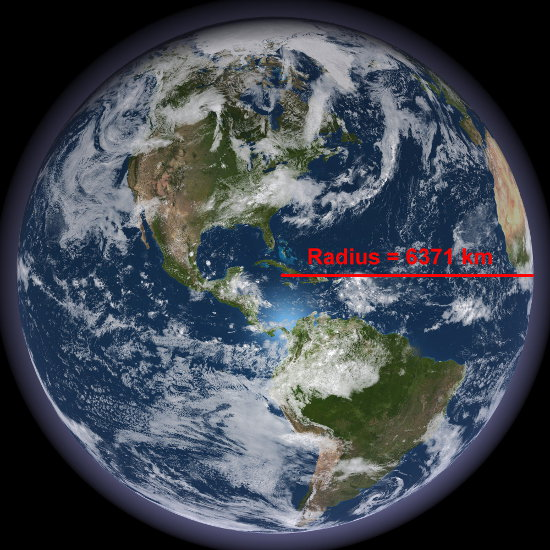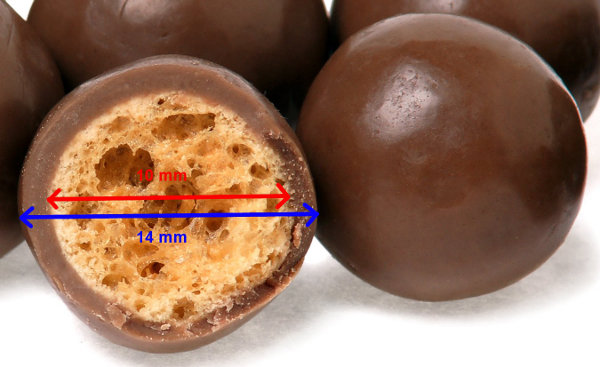# Volume Of Sphere

Volume of Sphere = 43 π r3

## Example One - Volume of Our PlanetAssume that our planet is round and has a radius of 6371 km. What is its volume?
(Use π = 3.14.)

Volume of sphere
= 43 π r3
= 43 × 3.14 × 6371 × 6371 × 6371
= 1 082 658 000 000 km3 approx.

## Example Two - Volume of Chocolate around a Malteser SweetA sweet called a Malteser is composed of a honeycomb sphere with a diameter of 10mm. The chocolate layer on the outside is 2 mm thick. What is the volume of chocolate coating on each Malteser? (Use π = 3.14.)

Radius of honeycomb = 5 mm
Radius of honeycomb and chocolate = 7 mm

Volume of honeycomb and chocolate
= 43 π r3
= 43 × 3.14 × 7 × 7 × 7
= 1436.03 mm3

Volume of honeycomb and chocolate
= 43 π r3
= 43 × 3.14 × 5 × 5 × 5
= 523.33 mm3

Volume of chocolate
= 1436.03 – 523.33
= 912.7 mm3

## Question - Volume of a Deep-Sea Submersible

Remote-controlled deep-sea submersibles are used to explore the ocean depths for mining companies. Metals such as silver, gold and copper can be found in greater concentrations than in terrestrial mines. Because the pressure of water crushing in from all sides is enormous, the submersible often has spherical ends for the external water pressure to be evenly distributed so that there is no weak spot. What is the volume inside a submersible with a diameter of 6 metres? (Use π = 3.14.)